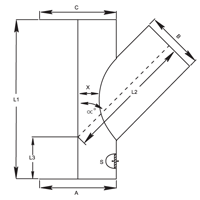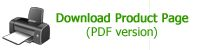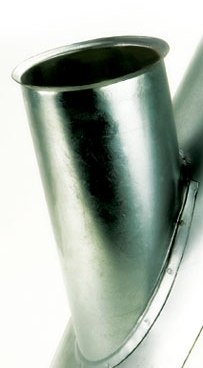### 45° Duct Entries

Duct entries allow a new run of Speedlock modular ducting to branch off your existing system - even if it is not Eximo speedlock ducting. Specify the diameter of the main duct, and the branch.

Code DE45### Dimensional Specifications

 Product Code A mm B mm C mm L1 mm L2 mm L3 mm ∞° S mm DE45 Choose ID 80 Choose ID 300 calculate calculate 43.80 0.90 DE45 Choose ID 100 Choose ID 300 calculate calculate 43.80 0.90 DE45 Choose ID 120 Choose ID 350 calculate calculate 44.00 0.90 DE45 Choose ID 125 Choose ID 350 calculate calculate 44.00 0.90 DE45 Choose ID 140 Choose ID 350 calculate calculate 44.10 0.90 DE45 Choose ID 150 Choose ID 400 calculate calculate 44.20 0.90 DE45 Choose ID 160 Choose ID 400 calculate calculate 44.20 0.90 DE45 Choose ID 180 Choose ID 400 calculate calculate 44.30 0.90 DE45 Choose ID 200 Choose ID 450 calculate calculate 44.40 0.90 DE45 Choose ID 225 Choose ID 500 calculate calculate 44.50 0.90 DE45 Choose ID 250 Choose ID 500 calculate calculate 44.50 0.90 DE45 Choose ID 275 Choose ID 600 calculate calculate 44.60 0.90 DE45 Choose ID 300 Choose ID 600 calculate calculate 44.60 0.90 DE45 Choose ID 315 Choose ID 600 calculate calculate 44.60 0.90 DE45 Choose ID 350 Choose ID 700 calculate calculate 44.70 0.90 DE45 Choose ID 400 Choose ID 800 calculate calculate 44.70 0.90 DE45 Choose ID 450 Choose ID 950 calculate calculate 44.70 0.90 DE45 Choose ID 500 Choose ID 1050 calculate calculate 44.80 0.90 DE45 Choose ID 550 Choose ID 1150 calculate calculate 44.80 0.90 DE45 Choose ID 600 Choose ID 1250 calculate calculate 44.80 0.90 DE45 Choose ID 650 Choose ID 1250 calculate calculate 44.80 0.90 DE45 Choose ID 700 Choose ID 1300 calculate calculate 44.80 0.90 DE45 Choose ID 750 Choose ID 1300 calculate calculate 44.80 0.90 DE45 Choose ID 800 Choose ID 1450 calculate calculate 44.80 0.90 DE45 Choose ID 850 Choose ID 1450 calculate calculate 44.90 0.90 DE45 Choose ID 900 Choose ID 1650 calculate calculate 44.90 0.90
`All product design specifications are subject to change without notice. `
`Images are for illustration purposes only`

Subscribe for a Complete CatalogueMaterial
0.9mm galvanised steel

Sizes
Manufactured to order

Dimensions
Customer to specify A, B & C. Please note that options are limited by the fact that
A = C & A ≥ B

The branch determines the length L1. The duct entry can only be fitted to straight duct with the branch placed centrally on the plate

If B < 300: X = 50mm
If B > 300: X = 100mmdeveloped by Pretty Clever Consulting

### Additional Product Information and Availability

Name

Company

Email

Product Diameter (optional)